# HSSlive: Plus One & Plus Two Notes & Solutions for Kerala State Board

## BSEB Class 10 Maths Chapter 13 Surface Areas and Volumes Ex 13.5 Textbook Solutions PDF: Download Bihar Board STD 10th Maths Chapter 13 Surface Areas and Volumes Ex 13.5 Book AnswersBSEB Class 10 Maths Chapter 13 Surface Areas and Volumes Ex 13.5 Textbook Solutions PDF: Download Bihar Board STD 10th Maths Chapter 13 Surface Areas and Volumes Ex 13.5 Book Answers

BSEB Class 10th Maths Chapter 13 Surface Areas and Volumes Ex 13.5 Textbooks Solutions and answers for students are now available in pdf format. Bihar Board Class 10th Maths Chapter 13 Surface Areas and Volumes Ex 13.5 Book answers and solutions are one of the most important study materials for any student. The Bihar Board Class 10th Maths Chapter 13 Surface Areas and Volumes Ex 13.5 books are published by the Bihar Board Publishers. These Bihar Board Class 10th Maths Chapter 13 Surface Areas and Volumes Ex 13.5 textbooks are prepared by a group of expert faculty members. Students can download these BSEB STD 10th Maths Chapter 13 Surface Areas and Volumes Ex 13.5 book solutions pdf online from this page.

## Bihar Board Class 10th Maths Chapter 13 Surface Areas and Volumes Ex 13.5 Books Solutions

 Board BSEB Materials Textbook Solutions/Guide Format DOC/PDF Class 10th Subject Maths Chapter 13 Surface Areas and Volumes Ex 13.5 Chapters All Provider Hsslive

## How to download Bihar Board Class 10th Maths Chapter 13 Surface Areas and Volumes Ex 13.5 Textbook Solutions Answers PDF Online?

2. Click on the Bihar Board Class 10th Maths Chapter 13 Surface Areas and Volumes Ex 13.5 Answers.
3. Look for your Bihar Board STD 10th Maths Chapter 13 Surface Areas and Volumes Ex 13.5 Textbooks PDF.
4. Now download or read the Bihar Board Class 10th Maths Chapter 13 Surface Areas and Volumes Ex 13.5 Textbook Solutions for PDF Free.

## BSEB Class 10th Maths Chapter 13 Surface Areas and Volumes Ex 13.5 Textbooks Solutions with Answer PDF Download

Find below the list of all BSEB Class 10th Maths Chapter 13 Surface Areas and Volumes Ex 13.5 Textbook Solutions for PDF’s for you to download and prepare for the upcoming exams:

## BSEB Bihar Board Class 10th Maths Solutions Chapter 13 Surface Areas and Volumes Ex 13.5

Question 1.
A copper wire 3 mm in diameter is wound about a cylinder whose length is 12 cm, and diameter 10 cm, so as to cover the curved surface of the cylinder. Find the length and mass of the wire, assuming the density of copper to be 8.88 g per cm³.
Solution:
Curved surface area of the cylinder
= 2πrh = 2 x 3.14 x 5 x 12 cm²
= 120 x 3.14 cm²
Diameter i.e., width of the wire
= 3 mm = 310 cm
Let length of the wire be l cm.
So, area of the wire = l x 310 cm²
So, l x 310 = 120 x 3.14
or l = 120×3.14×103 cm = 1256 cm
Now volume of wire = πr²h
= 3.14 x 320 x 320 x 1256 cm³
So, weight of wire = 3.14 x 9400 x 1256 x 8.88 g
= 789.98 g (approx.)

Question 2.
A right triangle, whose sides are 3 cm and 4 cm (other than hypotenuse) is made to revolve about its hypotenuse. Find the volume and surface area of the double cone so formed. (Choose value of n as found appropriate.)
Solution:
In the right angled ∆ ABC,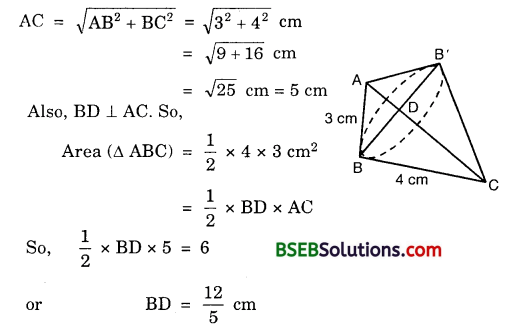So, the radius of the base for both the cones = 125 cm.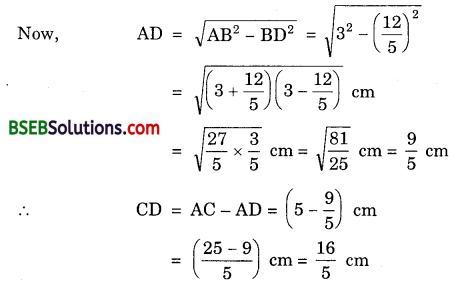∴ Volume of the cone ABB’
= 13 x 227 x 14425 x 95cm³ = 9504875 cm³
Volume of the cone CBB’
= 13π.BD².CD
= 13 x 227 x 14425 x 165cm³ = 16896875 cm³
∴Volume of the double cone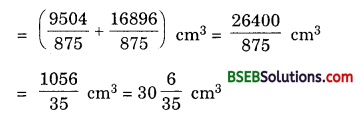Curved surface area of the cone ABB’
= π.BD.CD
= 227 x 125 x 3 cm² = 79235 cm²
Curved surface area of the cone CBB’
= π.BD.CD
= 227 x 125 x 4 cm² = 105635 cm²
∴ Surface area of the double cone =
= (79235+105635) cm²
= 184835 cm²
= 52.8 cm²

Question 3.
A cistern, internally measuring 150 cm x 120 cm x 110 cm, has 129600 cm³ of water in it. Porous bricks are placed in the water until the cistern is full of the brim. Each brick absorbs one-seventeenth of its own volume of water. How many bricks can be put in without overflowing the water, each brick being 22.5 cm x 7.5 cm x 6.5 cm?
Solution:
Volume of the cistern = 150 cm x 120 cm x 110 cm = 1980000 cm³
Let n bricks can be put in the cistern without over flowing water
Then, volume of n bricks = n x (22.5 x 7.5 x 6.5) cm³ = 1096.875 x n cm³
Volume of water absorbed by n bricks
= 117 x 1096.875 x n cm³
= 64.52 x n cm³
∴1096.875 x n + 129600 – 64.52 x n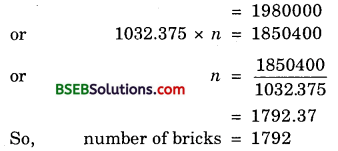Question 4.
In one fortnight of a given month, there was a rainfall of 10 cm in a river valley. If the area of the valley is 97280 km2, show that the total rainfall was approximately equivalent to the addition to the normal water of three rivers each 1072 km long, 75 m wide and 3 m deep.
Solution:
Volume of water in the 3 riversSo, the addition of water in the rivers is not equivalent to the rainfall.

Question 5.
An oil funnel of tin sheet consists of a 10 cm long cylindrical portion attached to a frustum of a cone. If the total height is 22 cm, diameter of the cylindrical portion is 8 cm and the diameter of the top of the funnel is 18 cm, find the area of the tin sheet required to make the funnel (see figure).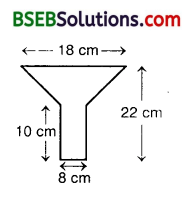Solution:
The diameter of the cylindrical portion is 8 cm.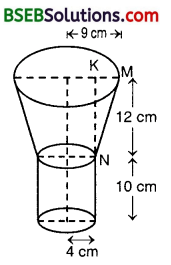Radius of top portion = 9 cm
Here, r = 4 cm, R = 9 cm, h = 12 cm
KM = (9-4) cm = 5 cm
∴ NM² = l² = KM² + KN2
= 5² + 12²
= 25 + 144 = 169
So, l = 169‾‾‾‾√ cm = 13 cm
Now, the tin required will be equal to the total surface area of the figure.
Area of the tin required = Curved surface area of the cylinder + Curved surface area of the frustumQuestion 6.
Derive the formula for the curved surface area and total surface area of the frustum of a cone, given to you in Section 13.5, using the symbols as explained.
Solution:
Curved surface area of the frustum RPQS = Curved surface area of the right circular cpne OPQ – Curved surface area of the right circular cone ORS
= πr1l1 – πr2l2 … (1)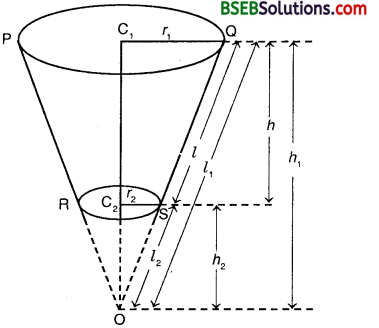Now, since ∆ OC1Q and ∆ OC2S are similar, thereforePutting the value of l1 in terms of r1, r2 and l2 in equation (1), we have :
Curved surface area of the frustum∴Total surface area of the frustum
= Curved surface area of the frustum + πr1² + πr2²
= π(r1 + r2)l + πr1² + πr2²
= π[(r1 + r2)l + r1² + r2²]

Question 7.
Dev ive the formula for the volume of the frustum of a cone, given to you in Section 13.5, using the symbols as explained.
Solution:
Volume of the frustum RPQS = Volume of the right circular cone OPQ – Volume of the right circular cone ORS
= 13πr1²h1 – 13πr2²h2
= 13π(r1²h1 – r2²h2) … (1)
Now, since ∆ OC1Q and ∆ OC2S are similar, therefore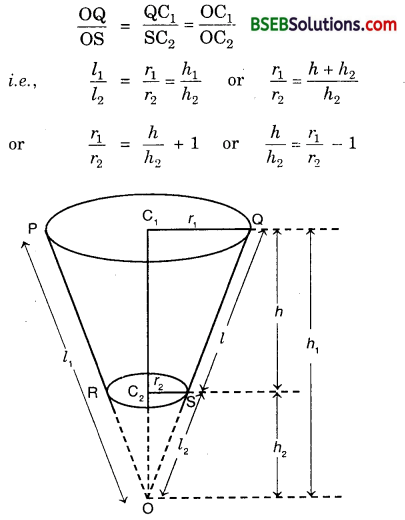or h1 = (𝑟1𝑟2−1) h2 = (𝑟1−𝑟2𝑟2)h2 … (2)
Putting the value of in terms of rr r2 and h2 in eqn. (1), we have :
Volume of the frustum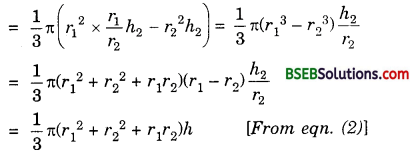## Bihar Board Class 10th Maths Chapter 13 Surface Areas and Volumes Ex 13.5 Textbooks for Exam Preparations

Bihar Board Class 10th Maths Chapter 13 Surface Areas and Volumes Ex 13.5 Textbook Solutions can be of great help in your Bihar Board Class 10th Maths Chapter 13 Surface Areas and Volumes Ex 13.5 exam preparation. The BSEB STD 10th Maths Chapter 13 Surface Areas and Volumes Ex 13.5 Textbooks study material, used with the English medium textbooks, can help you complete the entire Class 10th Maths Chapter 13 Surface Areas and Volumes Ex 13.5 Books State Board syllabus with maximum efficiency.

## FAQs Regarding Bihar Board Class 10th Maths Chapter 13 Surface Areas and Volumes Ex 13.5 Textbook Solutions

#### How to get BSEB Class 10th Maths Chapter 13 Surface Areas and Volumes Ex 13.5 Textbook Answers??

Students can download the Bihar Board Class 10 Maths Chapter 13 Surface Areas and Volumes Ex 13.5 Answers PDF from the links provided above.

#### Can we get a Bihar Board Book PDF for all Classes?

Yes you can get Bihar Board Text Book PDF for all classes using the links provided in the above article.

## Important Terms

Bihar Board Class 10th Maths Chapter 13 Surface Areas and Volumes Ex 13.5, BSEB Class 10th Maths Chapter 13 Surface Areas and Volumes Ex 13.5 Textbooks, Bihar Board Class 10th Maths Chapter 13 Surface Areas and Volumes Ex 13.5, Bihar Board Class 10th Maths Chapter 13 Surface Areas and Volumes Ex 13.5 Textbook solutions, BSEB Class 10th Maths Chapter 13 Surface Areas and Volumes Ex 13.5 Textbooks Solutions, Bihar Board STD 10th Maths Chapter 13 Surface Areas and Volumes Ex 13.5, BSEB STD 10th Maths Chapter 13 Surface Areas and Volumes Ex 13.5 Textbooks, Bihar Board STD 10th Maths Chapter 13 Surface Areas and Volumes Ex 13.5, Bihar Board STD 10th Maths Chapter 13 Surface Areas and Volumes Ex 13.5 Textbook solutions, BSEB STD 10th Maths Chapter 13 Surface Areas and Volumes Ex 13.5 Textbooks Solutions,
Share: